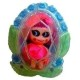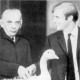# Gracious Living

Local Connectedness and Some Weird Counterexamples
November 10, 2010, 14:25
Filed under: Math, Topology | Tags: , , , , ,

Last time we talked about connectedness and path-connectedness and showed that though all path-connected spaces are also connected, connected spaces are not in general path-connected. There is a partial remedy to this problem, found in the local versions of the connectedness properties.  Recall that a local property says something about neighborhoods of each point, rather than the space itself or its open sets.  Below the fold, I talk about local connectedness and local path-connectedness, and bring up a couple examples and counterexamples, including one of my favorites, the Conway base-13 function.

A locally connected space is one in which every point has a basis of neighborhoods which are all connected.  A locally path-connected space is one in which every point has a basis of neighborhoods which are all path-connected.  As usual, it’s nice to restate these properties in other ways: a space is locally (path-)connected if and only if it has a basis of (path-)connected open sets.  This might seem baffling at first — where did the localness go? — but remember that we can get a basis of neighborhoods at a point from a basis by taking all the basis elements that contain that point, and we can go the other way by just taking the union of all the bases of neighborhoods.

What I’d like to show is that a connected and locally path-connected space is also path-connected.  It’s worth pointing out first that the topologist’s sine curve, our connected but not path-connected space, isn’t locally path-connected at any point on its vertical segment: since it has the subspace topology, neighborhoods of any such point contain pieces from infinitely many of its oscillations.  So this looks hopeful.  But there’s a deeper idea we can get at here: the components of a space.

Recall that a component is an equivalence class of points under the relation “two points are equivalent if a connected set contains them,” and ditto for path components.  These are basically the largest possible (path-)connected sets.  The topologist’s sine curve has one component and two path components: its vertical segment, and its curvy bit (you need to pass an infinite number of oscillations to get from one to the other, but to go between points on the curvy bit, you only need to pass a finite number of oscillations).  Last time we said that path-connected spaces were connected, but this is really just a special case of the more general statement: every path component of a space is a subset of a component.  In math, we like general statements!

Let’s go further.  Check that the closure of a connected set is connected: this means that components are closed.  On the other hand, they’re only necessarily open if there are finitely many of them:$\mathbb{Q}$ has a component for every point, but its points aren’t open sets.  On the other hand, path components don’t need to be closed at all: the topologist’s sine curve is the closure of its curvy bit!  (I actually think the name “topologist’s sine curve” usually just means the “curvy bit,” but its closure is more interesting.)  This example also shows that the closure of a path-connected set isn’t necessarily connected.

But if a space is locally path-connected, then its path components are open since they’re just unions of all the path-connected neighborhoods.  Since path components are equivalence classes, they also form a partition of the space.  But a partition of a space into open sets is just a separation of it!  And since the path components are connected, they give you the best separation, i. e. one that can’t be separated any further.  This is precisely the separation into components.  Thus, the path components of a locally path-connected space are its components.

Local connectedness isn’t quite as useful, but it does deal with things like$\mathbb{Q}$, which is everywhere disconnected but not discrete.  Every neighborhood of a point in a locally connected space contains a connected neighborhood of that point.  If the set is everywhere disconnected, then the only connected set containing that point is itself.  Thus, every point is open, and so the set is discrete.  So locally connected sets are everywhere disconnected iff they are discrete.  In general, components of a local connected space are unions of connected neighborhoods, hence open.  So a locally connected space is the topological disjoint union of its components.

Let’s look at some counterexamples!  #1 is the “ordered square”:$[0,1]\times [0,1]$ with the order topology given by the dictionary order, where$(a,b)\le (c,d)$ if$a\le c$ or$a=c$ and$b\le d$.  I think we’ve seen this before, though I don’t remember where.  Since every point has an interval around it, the set is connected and locally connected.  But it’s not locally path-connected: in fact, it has uncountably many path components!  Namely, each vertical line intersected with the square is a path-component.  This is because, to draw a path from$(a,b)$ to$(c,d)$, we’d have to go down every vertical line$x=v$ in the square for$a.  So this would be a function from $[0,1]$ to what amounts to an infinite distance, which can’t be continuous for reasons we’ll soon see.#2 is the “broom space”.  Start at the point$(0,0)$ and draw a line to every point$(1,1/n)$ for$n$ a natural number.  Also draw the line from$(0,0)$ to$(1,0)$.  Give it the subspace topology from$\mathbb{R}^2$.  There’s a path between any two points because we can go from one to the origin and then to the other.  But any neighborhood of$(1,0)$ (or any point on the horizontal line) contains pieces of infinitely many of the other lines, and if this neighborhood is small enough that it doesn’t contain$(0,0)$, all these pieces will be different path components of the neighborhood.  So those points don’t have path-connected bases of neighborhoods.  Evidently this counterexample depends on all the lines being joined at a single point.

#3 is the cofinite topology on the natural numbers — remember that open sets here are those whose complement is finite.  This is obviously connected — so obviously that it’s called “hyperconnected,” meaning that any two open sets intersect.  Similarly, it’s locally connected.  But it’s neither path-connected nor locally path-connected.  If a path hits the numbers$a,b,c,d,e,\dotsc$ we know the preimage of each has to be closed since its complement, the preimage of everything but that number, is open by continuity.  But then$[0,1]$ is the countable disjoint union of closed sets, at least some of which must be intervals.  The only way to do this is by letting$[0,1]$ itself be the only one in the union; proofs are, for example, here (read the comments for other viewpoints/try it yourself first!).  So the only paths in the set are constant functions.  This proves that the set isn’t connected or path-connected.

#4 is the usefulness of some of this.  Most spaces we deal with are locally path-connected, so it’s irrelevant whether we want to find components or path components.  Being able to do either can give us lots of insight on what the space looks like.  My favorite example is Smale’s paradox.  I don’t actually know the proof, but hope to someday, and then I’ll post about it here.  Basically, Stephen Smale was studying the space of oriented immersions of the sphere into$\mathbb{R}^3$.  An immersion is a smooth map, not necessarily injective (so the sphere can pass through itself), but where every point has a tangent plane.  This means no holes, sharp corners or weird cusps.  Smale proved that this space was path-connected using topology; because of the “oriented” part, the normal sphere and the inside-out sphere were counted differently.  So one of the consequences of Smale’s proof was that there was a “path of immersions” between a sphere and its inside-out version — that is, you could turn a sphere inside out without making a hole or a corner.  What’s weird is that this proof doesn’t produce an example of such a path, it just says one exists.  Years later, the first example was produced (by a blind mathematician, no less).  A short version of such an example is here, taken from the short film that starts here.

#5 is only tangentially related, but it’s just crazy.  Yesterday we proved that the image of a connected set under a continuous function was connected.  One corollary was the Intermediate Value Theorem, or IVT: if a real-valued function is continuous on$[a,b]$ with$f(a)=c,f(b)=d$, then it attains every value in$[c,d]$ (it hits every horizontal line in between$y=c$ and$y=d$).  The natural question is: is every function that does this continuous?  Surprisingly, John Conway discovered a function, called the “base-13 function,” that is discontinuous at every point but achieves every value on every interval.  It’s defined on$(0,1)$.  Write every number in base 13 using the digits 0,1,2,3,4,5,6,7,8,9,*,-,+.  The *,-,+ are digits here, not operators; treat them as$A,B,C$ if it confuses you.  As usual, there’s some ambiguity with e.g. 0.4+++…=0.5000…, so we avoid recurring + where possible.  The function is defined so that$f(x)=0$ unless$x$ ends in …+abc…*def… or …-abc…*def…, where all the letters are digits between 0 and 9.  (So after that last *, there are no more occurrences of +,-, or * .)  If$x$ does have this form, define$f(x)$ to be the base 10 number with abc… before the decimal point and def… after it, and positive if the number we started with had a + in base 13, negative if it had a -.  Now, given any number in$(0,1)$, we can find a real as close to it as we want that gets sent to any real number: start with some decimal, go to the fiftieth “tredecimal place” or however small you want, and change all the digits from then on to$\pm$abc*def…  This proves that the function is discontinuous and that it satisfies the IVT in an extreme fashion.  Try graphing it!  No, don’t.OMG I love your blog! ^^

Comment by Lily[…] differently on the other side.  Shortly after the Sphere Theorem was proved, Stephen Smale of sphere eversion fame showed that homeomorphisms couldn’t always be made into diffeomorphisms — indeed, […][…] So far we’ve seen two basic families of properties of topological spaces.  The connectedness axioms tell us in what ways it is possible to break our space apart into pieces.  The compactness axioms […]

Pingback by Countability Axioms « Gracious Living